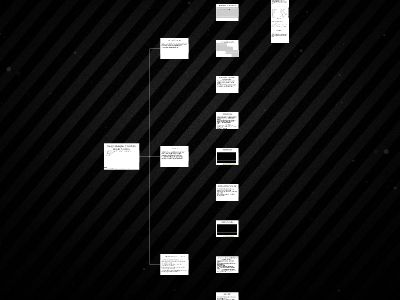Publications: 87 | Followers: 1

## Domain Knowledge and Function Composition

Publish on Category: Birds 0

Design Strategies 1: Combine Simpler Functions
CS 5010 Program Design Paradigms “Bootcamp”Lesson1.7
1
© Mitchell Wand,2012-2015This work is licensed under aCreativeCommons Attribution-NonCommercial4.0 International License.
Learning Objectives
At the end of this lesson, the student should be able to define short functions by composing existing functions.
2
Introduction
In this lesson, you will learn about Steps 4 and 5 of the design recipe: Design Strategies and Function Definitions.We will start with the simplest design strategy: Combine Simpler Functions
3
Programs are sets of Functions
We organize our programs as sets offunctions.A function takes an argument (or arguments) and returns a result.The contract says what kind of data the argument and result are.Purpose statement describes how the result depends on the argument.The design strategy is ashort description of how to get from the purpose statement to thecode.
4
Typical Program Design Strategies
5
Let's see where we are
6
Programs are sets of Functions
Design Strategy #1: Combine Simpler Functions
Many times the desired function can be described as a combination of simpler functions.This is what we did forf2c, where the simpler computations were just arithmetic.
7
Demo:velocity.rkt
On the next slide, you’ll see a video of me defining a function using the strategy “Combine Simpler Functions”.Observe how I followed the recipe: the contract, purpose statement, examples and tests were writtenbeforethe function definition.Oops:The contract should have saidReal, notNumber.The strategy should be “combine simpler functions” (we used to call this “function composition” but we decided to change it to a less fancy name.)The file is 01-4-velocity.rkt .
8
Demo:velocity.rkt
9
Note: you should never use Number when you mean Integer,NonNegInt, or Real. Here I should have used Real.
YouTube link
Another example: area-of-ring
Sometimes the simpler functions may include ones you write yourself.Here’s an example: area-of-ring, which calls area-of-circle.Both of these are defined by combining simpler functions.
10
Video: area-of-ring
11
I should have used Real (orNonNegReal) here, too.
YouTube link
What can you write in acombination of simpler functions?
Remember that the goal is to write beautiful programs.You want your reader to understand what you’re doing immediately.So just keep it simple.We won’t have formal rules about this, but:If the TA needs you to explain it, it’s not simple enough.Anything with anifis probably not simple enough.If you need anif, that’s a sign that you’re using a fancier design strategy. We’ll talk about these very soon.
12
Keep it short!
“Combining simpler functions” is for very short definitions only.If you’re writing something complicated, that means one of two things:You’re really using some more powerful design strategy (to be discussed)Your function needs to be split into simpler parts.If you have complicated stuff in your function you must have put it there for a reason. Turn it into a separate function so you can explain and test it.
13
When do you need to introduce new functions?
If a function has pieces that can be given meaningful contracts and purpose statements, then break it up and use function composition.Then apply the design recipe to design the pieces.
14
;; ball-after-tick : Ball -> Ball;; strategy: use template for Ball(define (ball-after-tick b)(if(and(<= YUP (where b) YLO)(or (<= (ball-x b) XWALL(+ (ball-x b)(ball-dxb)))(>= (ball-x b) XWALL(+ (ball-x b)(ball-dxb)))))(make-ball(- (* 2 XWALL)(ball-x (straight b 1.)))(ball-y (straight b 1.))(- (ball-dx(straight b 1.)))(ball-dy(straight b 1.)))(straight b 1.)))
;; ball-after-tick : Ball -> Ball;; strategy: combine simpler functions(define (ball-after-tick b)(if(ball-would-hit-wall? b)(ball-after-bounce b)(ball-after-straight-travel b)))
Here’s a pair of examples. Which do you think is clearer? Which looks easier to debug? Which would you like to have to defend in front of a TA?
15
Summary
In this lesson, you’ve learnedHow to use Function Composition to writea functiondefinition.When a function definition needs to be simplified by using help functions.How to use Cases to partition a scalar data type.
16
Next Steps
Study the filesIfyou have questions or comments about this lesson, post them on the discussion board.
17

0

Embed

Share

Upload

Make amazing presentation for free
Domain Knowledge and Function Composition